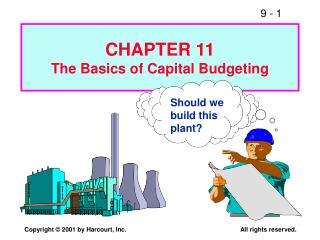DownloadDownload PresentationShould we build this plant?

Should we build this plant?

Télécharger la présentationShould we build this plant?

- - - - - - - - - - - - - - - - - - - - - - - - - - - E N D - - - - - - - - - - - - - - - - - - - - - - - - - - -
Presentation Transcript

1. CHAPTER 11The Basics of Capital Budgeting Should we build this plant?

2. What is capital budgeting? • Analysis of potential additions to fixed assets. • Long-term decisions; involve large expenditures. • Very important to firm’s future.

3. Steps 1. Estimate CFs (inflows & outflows). 2. Assess riskiness of CFs. 3. Determine k = WACC (adj.). 4. Find NPV and/or IRR. 5. Accept if NPV > 0 and/or IRR > WACC.

4. What is the difference between independent and mutually exclusive projects? Projects are: independent, if the cash flows of one are unaffected by the acceptance of the other. mutually exclusive, if the cash flows of one can be adversely impacted by the acceptance of the other.

5. An Example of Mutually Exclusive Projects BRIDGE vs. BOAT to get products across a river.

6. Normal Cash Flow Project: Cost (negative CF) followed by a series of positive cash inflows. One change of signs. Nonnormal Cash Flow Project: Two or more changes of signs. Most common: Cost (negative CF), then string of positive CFs, then cost to close project. Nuclear power plant, strip mine.

7. What is the payback period? The number of years required to recover a project’s cost, or how long does it take to get our money back?

8. Payback for Project L(Long: Large CFs in later years) 2.4 0 1 2 3 CFt -100 10 60 100 80 Cumulative -100 -90 -30 0 50 PaybackL = 2 + 30/80 = 2.375 years

9. Project S (Short: CFs come quickly) 1.6 0 1 2 3 CFt -100 70 100 50 20 Cumulative -100 -30 0 20 40 PaybackL = 1 + 30/50 = 1.6 years

10. Strengths of Payback: 1. Provides an indication of a project’s risk and liquidity. 2. Easy to calculate and understand. Weaknesses of Payback: 1. Ignores the TVM. 2. Ignores CFs occurring after the payback period.

11. NPV: Sum of the PVs of inflows and outflows.

12. What’s Project L’s NPV? Project L: 0 1 2 3 10% -100.00 10 60 80 9.09 49.59 60.11 18.79 = NPVL NPVS = \$19.98.

13. Rationale for the NPV Method NPV = PV inflows – Cost = Net gain in wealth. Accept project if NPV > 0. Choose between mutually exclusive projects on basis of higher NPV. Adds most value.

14. Using NPV method, which project(s) should be accepted? • If Projects S and L are mutually exclusive, accept S because NPVs > NPVL . • If S & L are independent, accept both; NPV > 0.

15. Internal Rate of Return: IRR 0 1 2 3 CF0 CF1 CF2 CF3 Cost Inflows IRR is the discount rate that forces PV inflows = cost. This is the same as forcing NPV = 0.

16. NPV: Enter k, solve for NPV. IRR: Enter NPV = 0, solve for IRR.

17. What’s Project L’s IRR? 0 1 2 3 IRR = ? -100.00 10 60 80 PV1 PV2 PV3 0 = NPV Enter CFs in CFLO, then press IRR: IRRL = 18.13%. IRRS = 23.56%.

18. Q. How is a project’s IRR related to a bond’s YTM? A. They are the same thing. A bond’s YTM is the IRR if you invest in the bond. 0 1 2 10 IRR = ? ... -1134.2 90 90 1090 IRR = 7.08% (use TVM or CFLO).

19. Rationale for the IRR Method If IRR > WACC, then the project’s rate of return is greater than its cost--some return is left over to boost stockholders’ returns. Example: WACC = 10%, IRR = 15%. Profitable.

20. IRR Acceptance Criteria • If IRR > k, accept project. • If IRR < k, reject project.

21. Decisions on Projects S and L per IRR • If S and L are independent, accept both. IRRs > k = 10%. • If S and L are mutually exclusive, accept S because IRRS > IRRL .

22. Reasons NPV different from IRR 1. Size (scale) differences. Smaller project frees up funds at t = 0 for investment. The higher the opportunity cost, the more valuable these funds, so high k favors small projects. 2. Timing differences. Project with faster payback provides more CF in early years for reinvestment. If k is high, early CF especially good, NPVS > NPVL.

23. Reinvestment Rate Assumptions • NPV assumes reinvest at k (opportunity cost of capital). • IRR assumes reinvest at IRR. • Reinvest at opportunity cost, k, is more realistic, so NPV method is best. NPV should be used to choose between mutually exclusive projects.

24. Managers like rates--prefer IRR to NPV comparisons. Can we give them a better IRR? Yes, MIRR is the discount rate that causes the PV of a project’s terminal value (TV) to equal the PV of costs. TV is found by compounding inflows at WACC. Thus, MIRR assumes cash inflows are reinvested at WACC.

25. MIRR for Project L (k = 10%) 0 1 2 3 10% -100.0 10.0 60.0 80.0 10% 66.0 12.1 10% MIRR = 16.5% 158.1 \$158.1 (1 + MIRRL)3 -100.0 TV inflows \$100 = PV outflows MIRRL = 16.5%

26. Why use MIRR versus IRR? MIRR correctly assumes reinvestment at opportunity cost = WACC. MIRR also avoids the problem of multiple IRRs. Managers like rate of return comparisons, and MIRR is better for this than IRR.